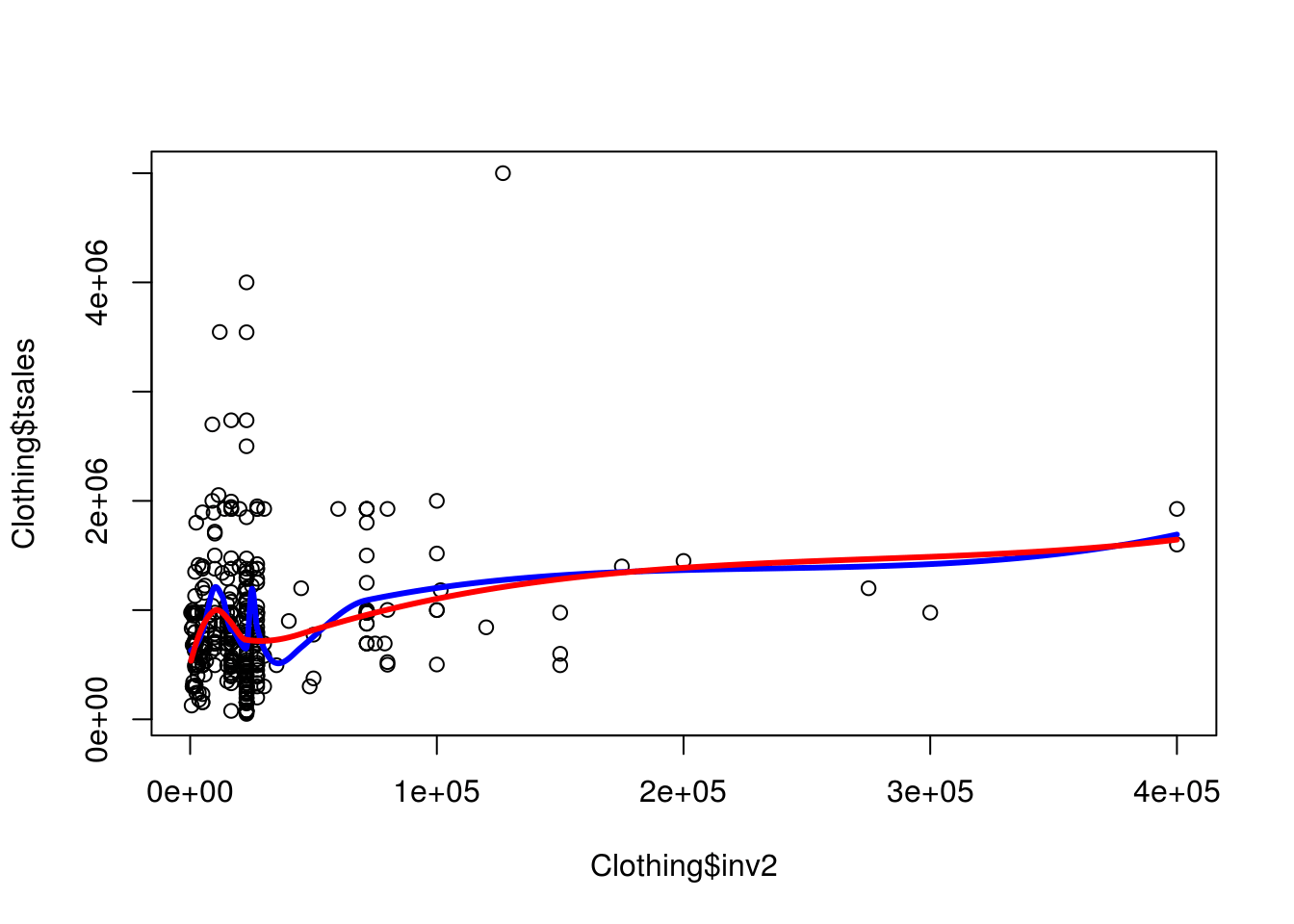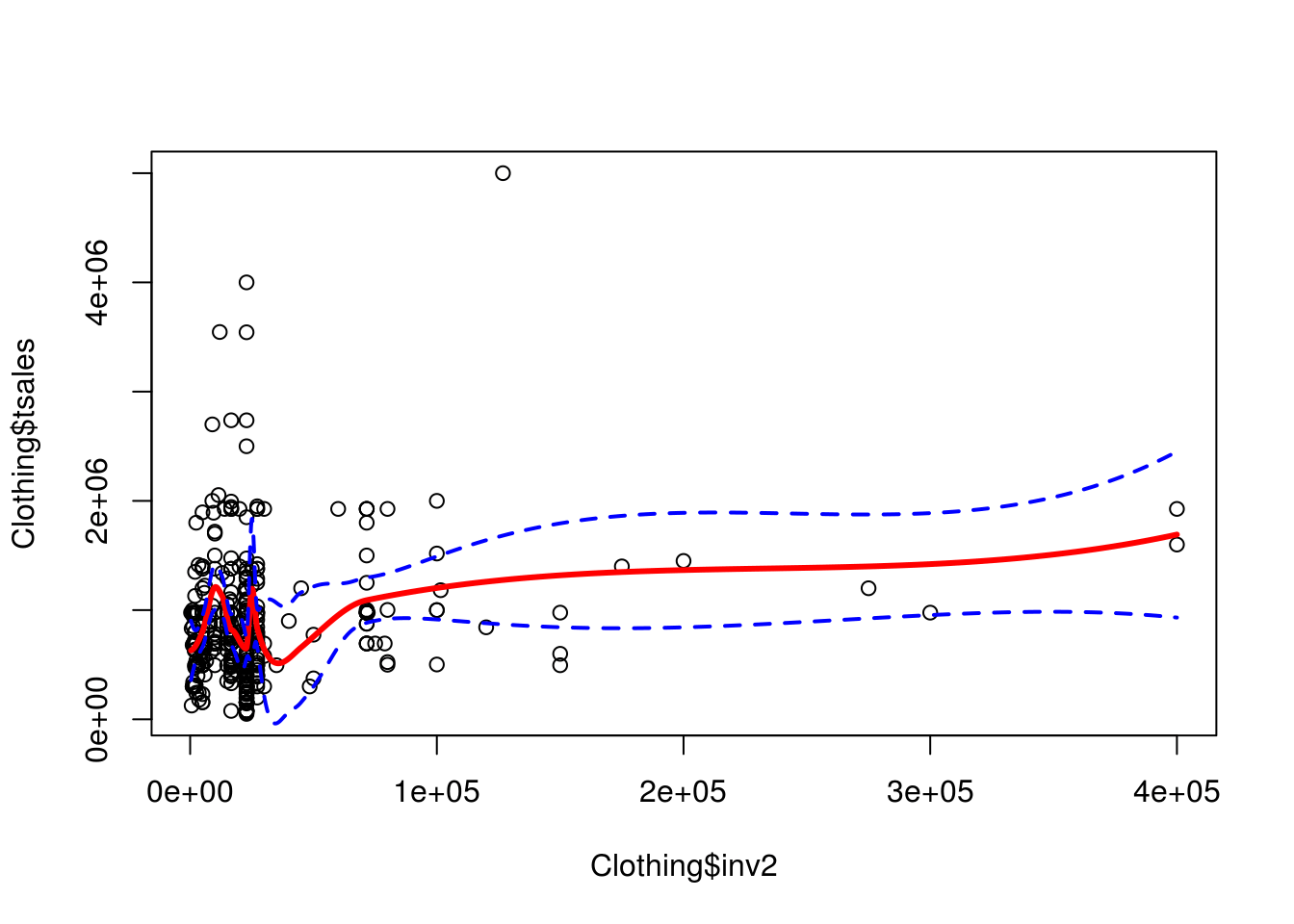# Local Regression in R

Local regression uses something similar to nearest neighbor classification to generate a regression line. In local regression, nearbyobservations are used to fit the line rather than all observations. It is necessary to indicate the percentage of the observations you want R to use for fitting the local line. The name for this hyperparameter is the span. The higher the span the smoother the line becomes.

Local regression is great one there are only a handful of independent variables in the model. When the total number of variables becomes too numerous the model will struggle. As such, we will only fit a bivariate model. This will allow us to process the model and to visualize it.

In this post, we will use the “Clothing” dataset from the “Ecdat” package and we will examine innovation (inv2) relationship with total sales (tsales). Below is some initial code.

``library(Ecdat)``
``````data(Clothing)
str(Clothing)``````
``````## 'data.frame':    400 obs. of  13 variables:
##  \$ tsales : int  750000 1926395 1250000 694227 750000 400000 1300000 495340 1200000 495340 ...
##  \$ sales  : num  4412 4281 4167 2670 15000 ...
##  \$ margin : num  41 39 40 40 44 41 39 28 41 37 ...
##  \$ nown   : num  1 2 1 1 2 ...
##  \$ nfull  : num  1 2 2 1 1.96 ...
##  \$ npart  : num  1 3 2.22 1.28 1.28 ...
##  \$ naux   : num  1.54 1.54 1.41 1.37 1.37 ...
##  \$ hoursw : int  76 192 114 100 104 72 161 80 158 87 ...
##  \$ hourspw: num  16.8 22.5 17.2 21.5 15.7 ...
##  \$ inv1   : num  17167 17167 292857 22207 22207 ...
##  \$ inv2   : num  27177 27177 71571 15000 10000 ...
##  \$ ssize  : int  170 450 300 260 50 90 400 100 450 75 ...
##  \$ start  : num  41 39 40 40 44 41 39 28 41 37 ...``````

There is no data preparation in this example. The first thing we will do is fit two different models that have different values for the span hyperparameter. “fit” will have a span of .41 which means it will use 41% of the nearest examples. “fit2” will use .82. Below is the code.

``````fit<-loess(tsales~inv2,span = .41,data = Clothing)
fit2<-loess(tsales~inv2,span = .82,data = Clothing)``````

In the code above, we used the “loess” function to fit the model. The “span” argument was set to .41 and .82.

We now need to prepare for the visualization. We begin by using the “range” function to find the distance from the lowest to the highest value. Then use the “seq” function to create a grid. Below is the code.

``````inv2lims<-range(Clothing\$inv2)
inv2.grid<-seq(from=inv2lims,to=inv2lims)``````

The information in the code above is for setting our x-axis in the plot. We are now ready to fit our model. We will fit the models and draw each regression line.

``````plot(Clothing\$inv2,Clothing\$tsales,xlim=inv2lims)
lines(inv2.grid,predict(fit,data.frame(inv2=inv2.grid)),col='blue',lwd=3)
lines(inv2.grid,predict(fit2,data.frame(inv2=inv2.grid)),col='red',lwd=3)``````Not much difference in the two models. For our final task, we will predict with our “fit” model using all possible values of “inv2” and also fit the confidence interval lines.

``````pred<-predict(fit,newdata=inv2.grid,se=T)
plot(Clothing\$inv2,Clothing\$tsales)
lines(inv2.grid,pred\$fit,col='red',lwd=3)
lines(inv2.grid,pred\$fit+2*pred\$se.fit,lty="dashed",lwd=2,col='blue')
lines(inv2.grid,pred\$fit-2*pred\$se.fit,lty="dashed",lwd=2,col='blue')``````Conclusion

Local regression provides another way to model complex non-linear relationships in low dimensions. The example here provides just the basics of how this is done is much more complicated than described here.